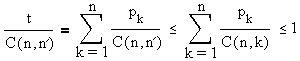# Sperner's Theorem

We know that if more than a half of subsets of an n-set A have been selected, there are bound to be at least two of which one contains another. This is proven with the help of the Pigeonhole Principle. Below we prove by far a stronger result - the Sperner's Theorem. It appears that a half is a few too many; there is no need to take this many subsets.

A collection B of nonempty subsets of a set A is called an antichain (or clutter or Sperner system) in A if no set in B is (properly) contained in another set from B.

Prove that, for an n-set A, the number of sets in any antichain in A cannot exceed C(n, n'), where n' = [(n + 1)/2].

To remind, C(n, m) is a binomial coefficientthat appears in the Binomial Theorem which, for an integer exponent, can be written as

(x + y)n = C(n,0)xn + C(n,1)xn-1y + C(n,2)xn-2y2 + ... + C(n,n)yn

For an even n, there are an odd number of binomial coefficients with the middle coefficient C(n, n') corresponding to n' = n/2. For n odd, the number of coefficients is even and there are two equal coefficients, one corresponding to n' = (n - 1)/2 and the other to n' = (n + 1)/2. For even n, [(n + 1)/2] = n/2, where [] means "the integer part".

At this time, I shall assume the unimodal behavior of the sequence of binomial coefficients: C(n, k) is not decreasing as k changes from k = 0 to k = n' and is not increasing afterwards. Therefore, for any n, C(n, n'), with n' = [(n + 1)/2] is the largest binomial coefficient:

For all 0 ≤ k ≤ n, C(n, k) ≤ C(n, n').

C(n, k) is the number of ways to select k (unordered) elements out of n. It follows that the number of sets in any antichain in which all sets have the same number of elements is bounded above by C(n, n').

A maximal chain in the n-set A is a sequence X0, X1,... ,Xn of subsets of A such that for all i card(Xi), cardinality or the number of elements in the set Xi, is i; and Xi ⊂ Xi+1, for i = 0, ..., n - 1. Obviously, there is a 1-1 correspondence between permutations of the set A and its maximal chains: any permutation {x1 x2 ... xn} gives rise to a maximal chain

Ø ⊂ {x1} ⊂ {x1, x2} ⊂ ... ⊂ {x1, x2, ..., xn} = A

and vice versa. Let B = {B1,B2,...,Bt} be an antichain in A, with card(Bi) = bi, i = 1, ..., t. By definition, any chain in A - maximal or not - can contain at most one of the sets Bi. Now, there are bi! ways of forming a chain from Ø to Bi, and there are (n - bi)! ways of extending this chain to a maximal one. Thus the number of maximal chains that contain the set Bi is bi!(n - bi)!. We therefore have the inequalitySuppose that among the cardinalities {bi} there are p1 1s, p2 2s, ..., pn n's. So that pk's are nonnegative integers that sum up to t. The previous inequality can be rewritten asThis is known as the Lubell-Yamamoto-Meshalkin (LYM) inequality. From here it follows at once thator t ≤ C(n, n').

## References

1. V.K. Balakrishnan, Theory and Problems of Combinatorics, Schaum's Outline Series, McGraw-Hill, 1995